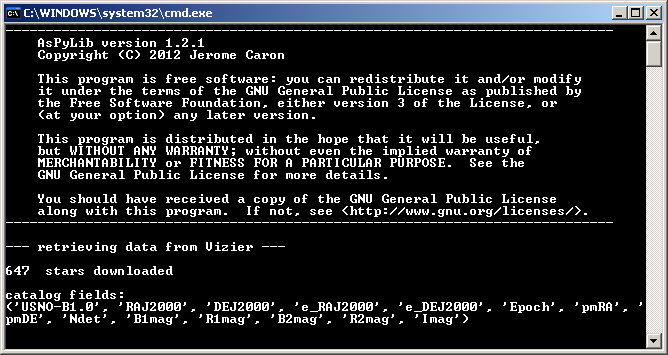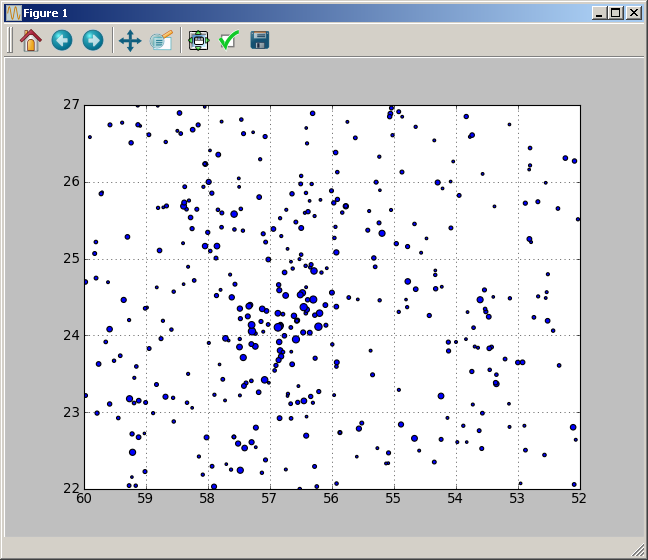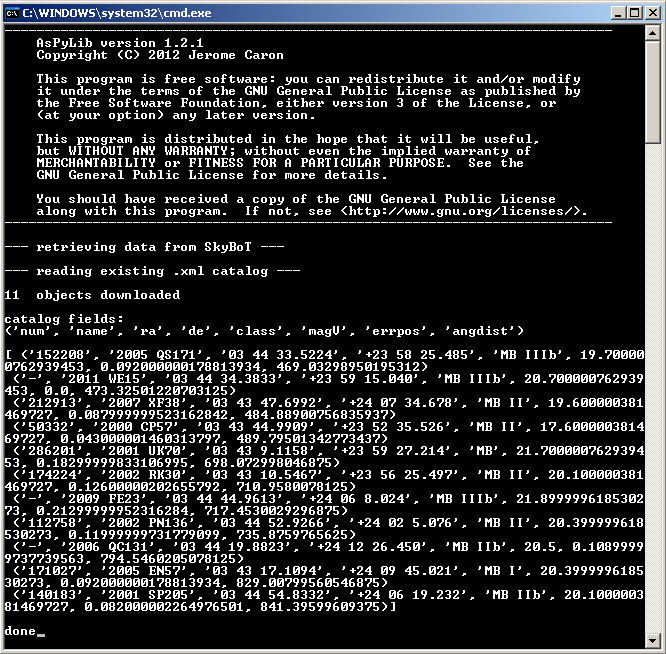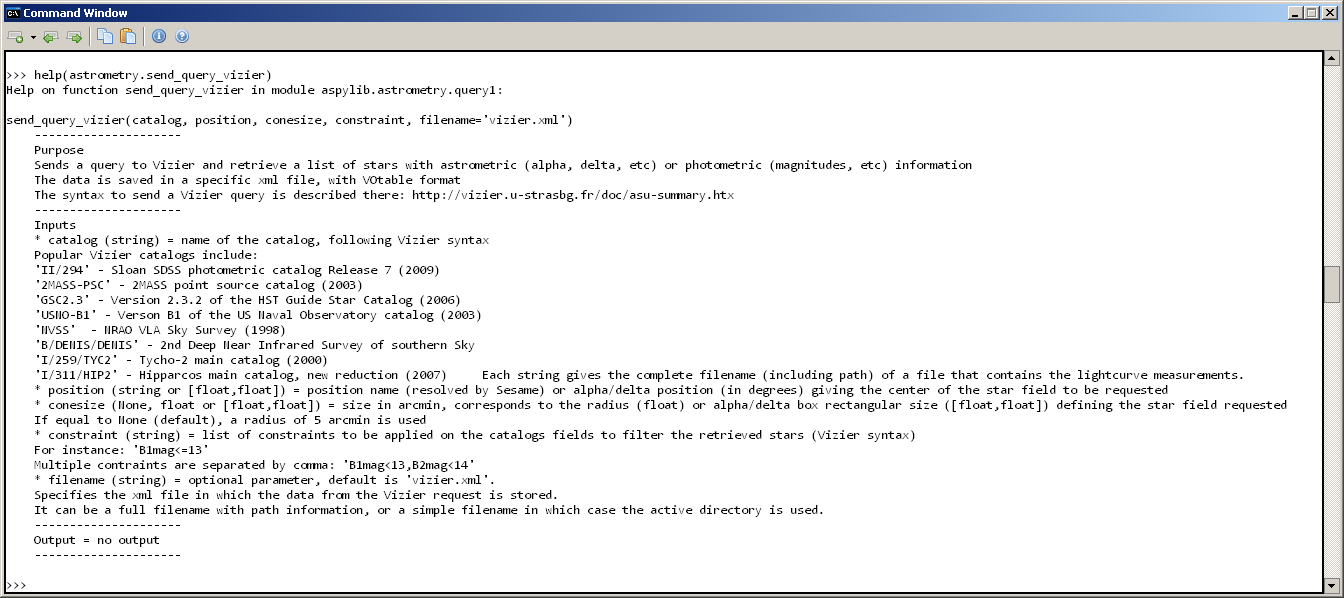# 7.1 Sending queries to web services (Vizier, SkyBoT)¶

## Retrieving data from Vizier¶

AsPyLib allows to send a simple “cone search” request to the Vizier service, and retrieve star positions and information from any catalog. To do it you only need to specify:

• a catalog
• a position (alpha, delta)
• a cone radius in arcmin, or a rectangular box size in arcmin x arcmin

To see how it works, the simplest way is to show an example. The script below retrieves all stars with magnitude smaller than 11, in an area of 7deg*10deg centered on the Pleiades, in Taurus.

```from aspylib import astrometry, astro
import matplotlib.pyplot as plt

#------ retrieving information from Vizier ------
catalog = 'USNO-B1'
position = [56., 24.]           #[alpha, delta] in degrees
conesize = [600., 420.]         #[boxsize_alpha, boxsize_delta] in arcmin
constraint = 'B1mag<=11'

astrometry.send_query_vizier(catalog, position, conesize, constraint)

#------ printing information --------------------
print
print
print "catalog fields:"
print stars.dtype.names

#------ generating sky map ----------------------
plt.figure()
plt.scatter(stars['RAJ2000'],stars['DEJ2000'],s=4*(12-stars['B1mag']))
plt.grid()
plt.xlim([52,60])
plt.ylim([22,27])
ax = plt.gca()
ax.set_xlim(ax.get_xlim()[::-1])
plt.show()
```

Then we get the following messages in the Python console:And the following sky map is shown:The first instruction sends a request to Vizier, and saves the returned VOtable file under the name “vizier.xml”

```astrometry.send_query_vizier(catalog, position, conesize, constraint)
```

The filename may be changed, as follows:

```astrometry.send_query_vizier(catalog, position, conesize, constraint, filename = "data.xml")
```

Then the second instruction opens the file, and convert it to a Numpy record array. Numpy record arrays are special arrays that allow to store columns with different data type, with a name and format for each column. They are discussed here for instance.

```stars = astrometry.read_votable("vizier.xml")
```

The name of the columns can be obtained with:

```print stars.dtype.names
```

And the data in a given column:

```print stars['column name']
```

## Retrieving data from SkyBoT¶

Sending queries to SkyBoT is pretty much the same. An example of script is given below:

```from aspylib import astrometry

#------ retrieving information from SkyBoT ------
date = 2455923.0
position = [56., 24.]           #[alpha, delta] in degrees
conesize = [30., 30.]           #[boxsize_alpha, boxsize_delta] in arcmin

astrometry.send_query_skybot(date,position,conesize)

#------ printing information --------------------
print
print
print "catalog fields:"
print cat.dtype.names
print
print cat
print
raw_input("done")
```

Then the following messages are printed in the Python console:Getting more information about the AsPyLib functions is quite easy thanks to the console help. Just by typing this:

```help(astrometry.send_query_vizier)
```

we get the following: